• Shuffle
Toggle On
Toggle Off
• Alphabetize
Toggle On
Toggle Off
• Front First
Toggle On
Toggle Off
• Both Sides
Toggle On
Toggle Off
Toggle On
Toggle Off
Front

### How to study your flashcards.

Right/Left arrow keys: Navigate between flashcards.right arrow keyleft arrow key

Up/Down arrow keys: Flip the card between the front and back.down keyup key

H key: Show hint (3rd side).h key

A key: Read text to speech.a keyPlay buttonPlay buttonProgress

1/35

Click to flip

### 35 Cards in this Set

• Front
• Back
 FDPs - Decimals Rounding to the nearest place value: If it is a 5 or greater, round it... UPIf not, keep the original value. FDPs - Decimals Decimal operations: what is 10 – 0.063? The key is to add zeroes to the bigger number to avoid confusions: 10.000 – 0.063. Now they have the same length and it is easier to do the subtraction FDPs - Decimals1.4 * 0.02 = ? ignore decimal point until the end. Multiply as if numbers were whole. Then, count the number of digits to the right of the decimals in both numbers and apply it to the product. 14 x 2 = 28, and we had 1 decimal on 1.4 and 2 decimals on 0.02, so number is 0.028 The same rules apply for division FDPs - Powers and Roots (0.5)³ = ?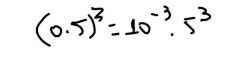Always use the integer. If necessary, convert the decimal into powers of ten. 0.125 FDPs - Powers and Roots ³√0.000027 = ?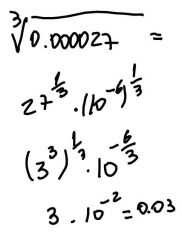Always use the integer. If necessary, convert the decimal into powers of ten. 0.03 FDPs - Powers and Roots (0.04)³ = ? Shortcut: the number of decimal places in the result of an x-numbered decimal x times the number of decimals of the original decimal: 0.04 * 0.04 * 0.04 = 0.000064³√0.000000008 = 0.002. A number with 9 decimals cube-rooted yields a number with 3 decimals. FDPs - Fractions If you add the same number to both the numerator and denominator of a fraction, the fraction gets closer to...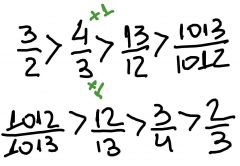1regardless of its original value. This means a proper fraction will increase its value, while an improper fraction will decrease. FDPs - FractionsTo divide fractions, use the... and then...If you see double decker fractions (1/2/3/4), this is the same as... use the RECIPROCAL and then MULTIPLY1/2 * 4/3 = 2/3 FDPs - Fractions To compare fractions, ....... and then ........... the numerators7/9 or 4/5?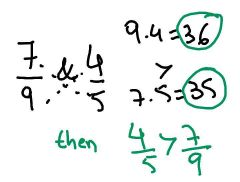CROSS-MULTIPLY and then COMPARE the numerators. FDPs - Percents “what is 30% of 80?” “75% of what number is 21?” “90 is what percent of 40?”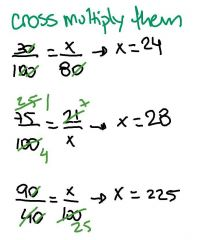Cross Multiply techinique FDPs - PercentsIf a quantity is increased by x percent, then the new quantity is (100 + x)% of the original. Thus, to find a 15% increase, multiply by 1.15 FDPs - Percents If a quantity is decreased by x percent, then the new quantity is (100 – x)% of the original. To find a 15% decrease, multiply by 0.85 FDPs - Percents By what percent did the price of the book increase? (1) The ratio original to new is 4:5 (2) the ratio change to new is 1:5 Both are sufficient, as this is the only information needed. FDPs - Successive percents A price which is increased by x% and then decreased by y% ORIGINAL . (1 + x%) . (1 – y%)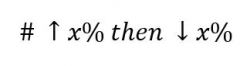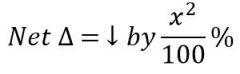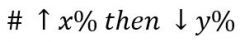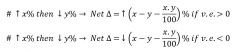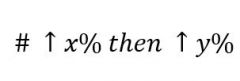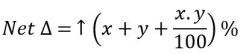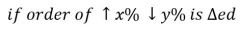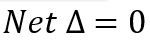The result remain unchanged What is the ultimate formula for Interest Rate?P - Principal / c = composition / t = time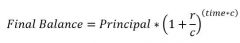Usually better to calculate simple interest + smt bigger FDPs - Advanced Terminating decimals only have 2’s and 5’s as ..... in their denominators.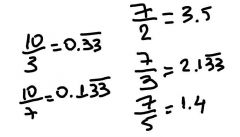prime factors FDPs - Unknown digit problems What are the step by step to deal with Unkown digit Problems? (1) Look at the answer choices first, to limit your search(2) use other given constraints to rule out additional possibilities(3) Focus on the units digit in the product or sum.(4) Test the remaining choices FDPs - Unknown digit problemsAB x CA = DEBC. In this multiplication, each letter stands for a different non-zero digit, and A.B < 10; What is AB? (A) 23 (B) 24 (C) 25 (D) 32 (E) 42 We can rule out choice C, as 2.5 = 10. Testing the others will result on E being the correct alternative.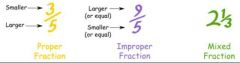FDPs - Fractions and Exponents and Roots Negative improper fractions when raised to an odd exponent will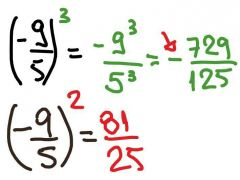will also yield a smaller number. FDPs - Fractions and Exponents and Roots All other fractions when raised to a power willyield a bigger number FDPs - Percents and weighted averages Cereal K is 10% sugar and Cereal B is 2% sugar. What should be the ratio of them to produce a 4% sugar cereal?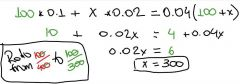Pick a smart number for one of the quantities and call the other quantity x. For example, picking 100 grams for Cereal K FDPs - Percents and weighted averages The revenue from pen sales went up 5%, but the revenue from pencil sales declined 13%. If the overall revenue was down 1%, what was the ratio of pen and pencil revenues? 100*1.05 + (1-0.13)x = (1-0.01)(100 + x) 6 = 0.12x x = 50So, the ratio is 2:1 FDPs - Percents and weighted averages A store sold 300 hammers and 12,000 nails on year 0 and 375 hammers and 18,000 nails on year 1. By approximately what percent did the ratio of hammers sold to nails sold decrease?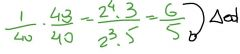Therefore, the decrease was from 6 to 5, or 1/6 (16.7%) FDPs - Estimating decimal equivalents Estimate 9/52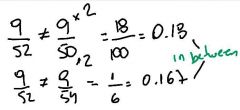9/52 is very close to the middle point of 0.167 and 0.18, which is approximately 0.173 When # between 0 & 1 is squared, they become... When # > 1 is squared, they become... ...SMALLER0 < x < 1 ; x² < x ....BIGGERx>1 ; x² > x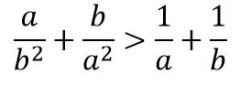When the following equation is true?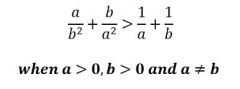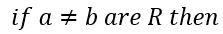The square of a + the square of b is > 2*a*b?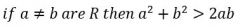Yes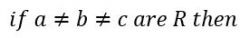a^2+b^2+c^2 > ab+bc+ca?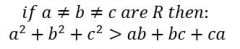Yes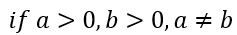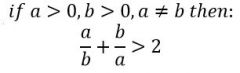0 = 1 when We say they are Perfect squaresWhen we compare x^2 to x^n We are dealing with a repeating decimal in this question. It's helpful to know that there's a way to write these kinds of decimals as a fraction. For example, the repeating decimal 0.444444444(4) may be written as 4949. So, 5959, 7979 and 8989 will all be repeating decimals. You might check it in your calculator. In order to make two decimal points repeat, you have to divide the two digit number by 99. For example, 2399=0.232323232323(23)2399=0.232323232323(23). Similarly, 3699=411=0.36363636(36)3699=411=0.36363636(36). Now it's clear that the minimum value of m=4m=4.